How Cheenta works to ensure student success?
Explore the Back-Story

# Test of Mathematics Solution Subjective 17 - Odd Coefficients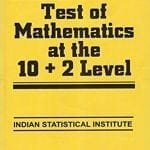This is a Test of Mathematics Solution (from ISI Entrance). The book, Test of Mathematics at 10+2 Level is Published by East West Press. This problem book is indispensable for the preparation of I.S.I. B.Stat and B.Math Entrance.

## Problem

If the coefficients of a quadratic equation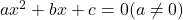, are all odd integers, show that the roots cannot be rational.

## Discussion

Suppose m and n are the roots. Then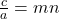.

Hence if one of them is rational then the other one is rational as well.

If possible say both roots are rational. Then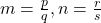where p,q,r,s are integers and g.c.d. (p,q) = 1, g.c.d.(r, s) = 1

Also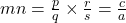.

Note that both r, s cannot be even (as their g.c.d. is 1). If possible say s is even, then r is odd. Also if s is even, p has to be even (since the 2's in s must be canceled out, as the product of the two fractions ultimately iswhich has odd in the numerator and odd in the denominator). Since p is even, therefore, q must be odd.

So here are the cases:

Case 1: p is even (say = 2p', q is odd, r is odd, s is even (say = 2s') (and other similar cases where crosswise numbers are even and other pair being odd)

Then.

This implies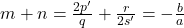.

Or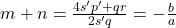.

Since qr is odd and 4s'p' is even their sum is odd. But the denominator (2s'p) is even. Hence the numerator cannot cancel the 2's in the denominator. But that is a contradiction as this fraction is supposed to be equal to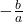where denominator is odd.

Case 2: all of p,q,r,s are odd.

Here the numerator is ps+qr which is even (since p, q, r, s are odd). But the denominator qs is odd. Hence 2's in the numerator won't cancel out. Hence contradiction.

Thus the roots cannot be rational.

## Chatuspathi

• What is this topic: Theory of Equations
• What are some of the associated concepts: Sum and product of roots, Vieta's formula
• Book Suggestions: Theory of Equations by Burnside and PantonThis is a Test of Mathematics Solution (from ISI Entrance). The book, Test of Mathematics at 10+2 Level is Published by East West Press. This problem book is indispensable for the preparation of I.S.I. B.Stat and B.Math Entrance.

## Problem

If the coefficients of a quadratic equation, are all odd integers, show that the roots cannot be rational.

## Discussion

Suppose m and n are the roots. Then.

Hence if one of them is rational then the other one is rational as well.

If possible say both roots are rational. Thenwhere p,q,r,s are integers and g.c.d. (p,q) = 1, g.c.d.(r, s) = 1

Also.

Note that both r, s cannot be even (as their g.c.d. is 1). If possible say s is even, then r is odd. Also if s is even, p has to be even (since the 2's in s must be canceled out, as the product of the two fractions ultimately iswhich has odd in the numerator and odd in the denominator). Since p is even, therefore, q must be odd.

So here are the cases:

Case 1: p is even (say = 2p', q is odd, r is odd, s is even (say = 2s') (and other similar cases where crosswise numbers are even and other pair being odd)

Then.

This implies.

Or.

Since qr is odd and 4s'p' is even their sum is odd. But the denominator (2s'p) is even. Hence the numerator cannot cancel the 2's in the denominator. But that is a contradiction as this fraction is supposed to be equal towhere denominator is odd.

Case 2: all of p,q,r,s are odd.

Here the numerator is ps+qr which is even (since p, q, r, s are odd). But the denominator qs is odd. Hence 2's in the numerator won't cancel out. Hence contradiction.

Thus the roots cannot be rational.

## Chatuspathi

• What is this topic: Theory of Equations
• What are some of the associated concepts: Sum and product of roots, Vieta's formula
• Book Suggestions: Theory of Equations by Burnside and Panton

### Knowledge Partner